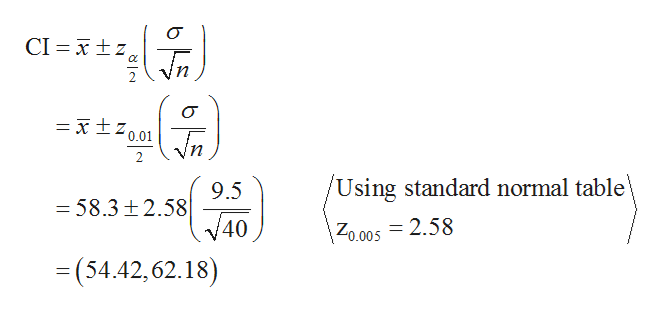# 11) Randomely selected students participated in an experiment to test their ability to determine when one minute (60 seconds) has passed. Forty students yielded a a sample mean of 58.3 seconds. Assume that σ = 9.5 seconds.What is the best point estimate for the mean for all student times?Construct a 99 % confidence interval estimate for the population mean for all stu- dents.Is it likely that their mean have an estimate that is reasonably close to 60 seconds?12) What sample size is needed to estimate the mean white blood cell count for the population of adults in the U.S? Assume you want a 99% confidence that the sample mean is within .2 of the population mean. The population standard deviation is 2.5.

Question
148 views

11) Randomely selected students participated in an experiment to test their ability to determine when one minute (60 seconds) has passed. Forty students yielded a a sample mean of 58.3 seconds. Assume that σ = 9.5 seconds.
What is the best point estimate for the mean for all student times?

Construct a 99 % confidence interval estimate for the population mean for all stu- dents.

Is it likely that their mean have an estimate that is reasonably close to 60 seconds?

12) What sample size is needed to estimate the mean white blood cell count for the population of adults in the U.S? Assume you want a 99% confidence that the sample mean is within .2 of the population mean. The population standard deviation is 2.5.

check_circle

Step 1

Hey, since there are multiple questions posted, we will answer first question. If you want any specific question to be answered then please submit that question only or specify the question number in your message.

Step 2

It is given that the sample mean is 58.3, sample size n is 40 and population standard deviation is 9.5.

The best point estimate for the mean for all student times is sample mean. Therefore, the best point estimate for all student tim...help_outlineImage TranscriptioncloseCI xtz x10.01 n /Using standard normal table 9.5 -58.3 t2.58 40 Zo.005 = 2.58 =(54.42,62.18) fullscreen

### Want to see the full answer?

See Solution

#### Want to see this answer and more?

Solutions are written by subject experts who are available 24/7. Questions are typically answered within 1 hour.*

See Solution
*Response times may vary by subject and question.
Tagged in

### Statistics